# Change total points for an assignment

You can change the total points for an assignment using the Assignment Editor.

NOTE: If you design a lesson for your students to submit for grades (similar to an assignment), each question within the lesson is automatically worth one point of the total and can't be edited. Example — A lesson with four questions in it will automatically have a total point value of 4.

The total points for an assignment is changed by editing the point value for each individual question within the assignment.

These edited point values for each question are then summed to give a total point value for the assignment.

The assignment total point value is visible in the:

• Assignment Editor (while creating or editing the assignment)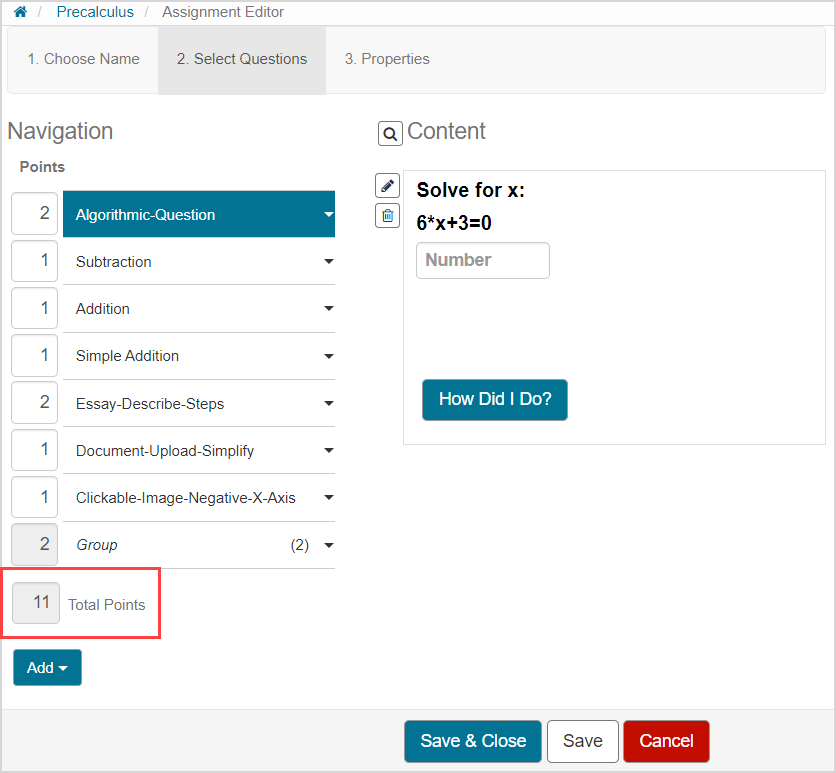• Gradebook (while viewing the graded assignment as a student or instructor)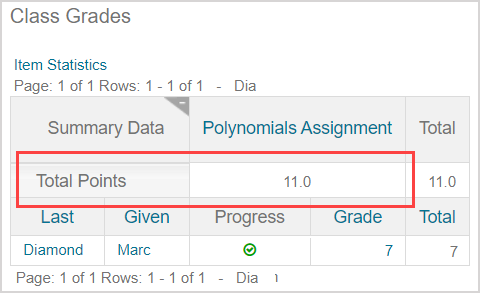## To change the total points for an assignment

To change the total points for an assignment:

1. Access the assignment that you want to edit the total points for using the Assignment Editor.

TIP: This assignment could be an assignment you are currently creating using the Assignment Editor (check out Create an assignment), or an assignment that already exists in your Content Repository that you want to edit using the Assignment Editor (check out Edit an assignment).

NOTE: The total points for an assignment with active attempts can't be edited until all active attempts are submitted or the assignment is force graded. Check out Force grade an activity.

1. Navigate to the 2. Select Questions tab of the assignment's Assignment Editor.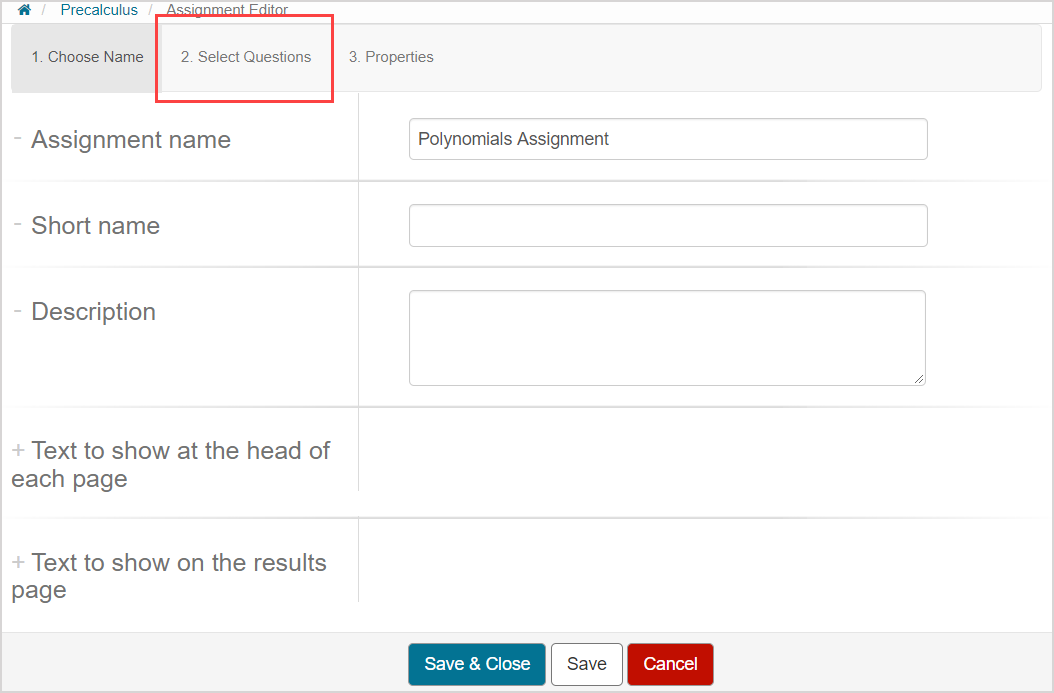1. Click on the Points field for each question and edit the numerical value for each question.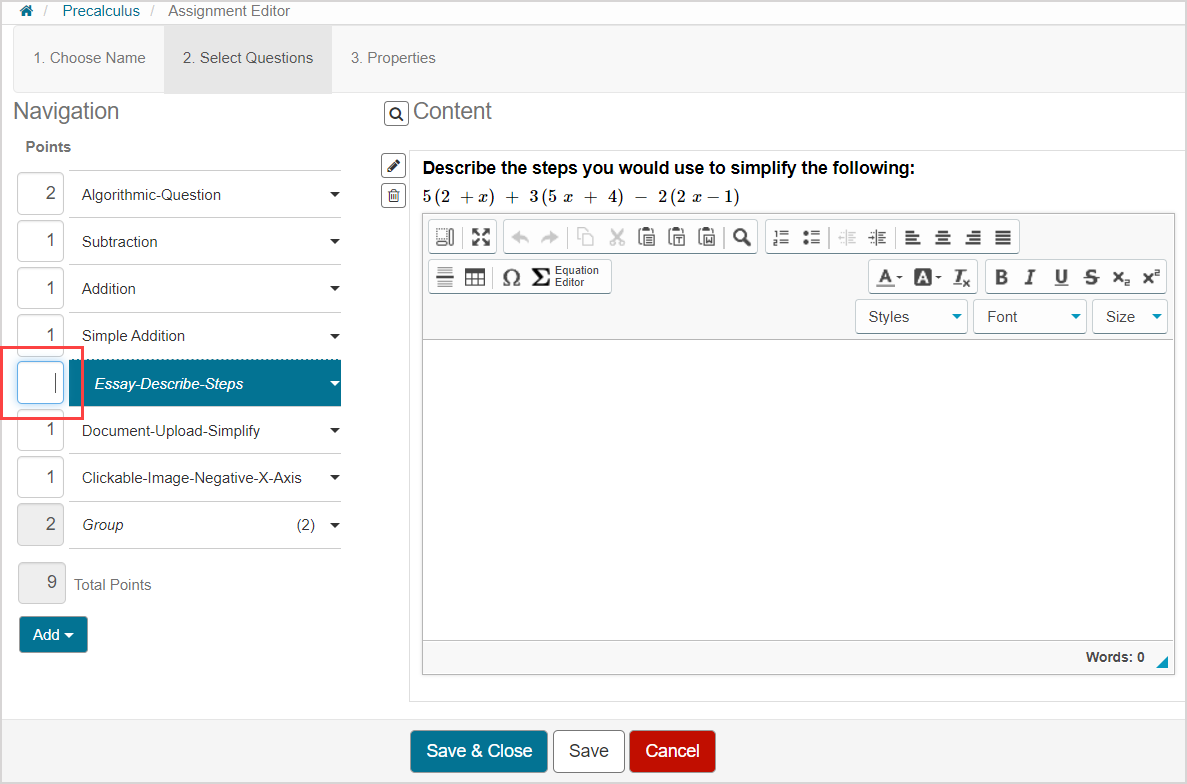TIP: Check out the Define the point value of a question group section in the Create a question group for an assignment topic to learn how to edit the Points field for a question group.

1. Press Enter on your keyboard (or click outside the question's Points field) for the assignment's Total Points field to update with the new total points value.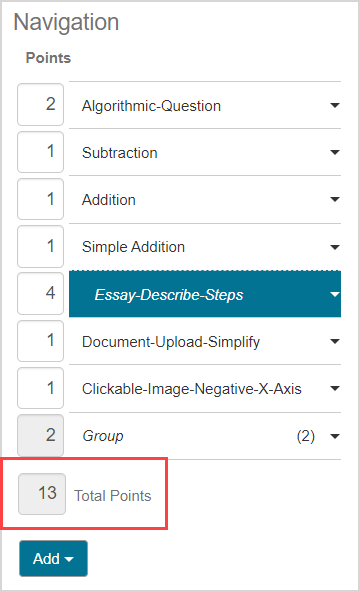1. Click Save to save your new assignment total points value.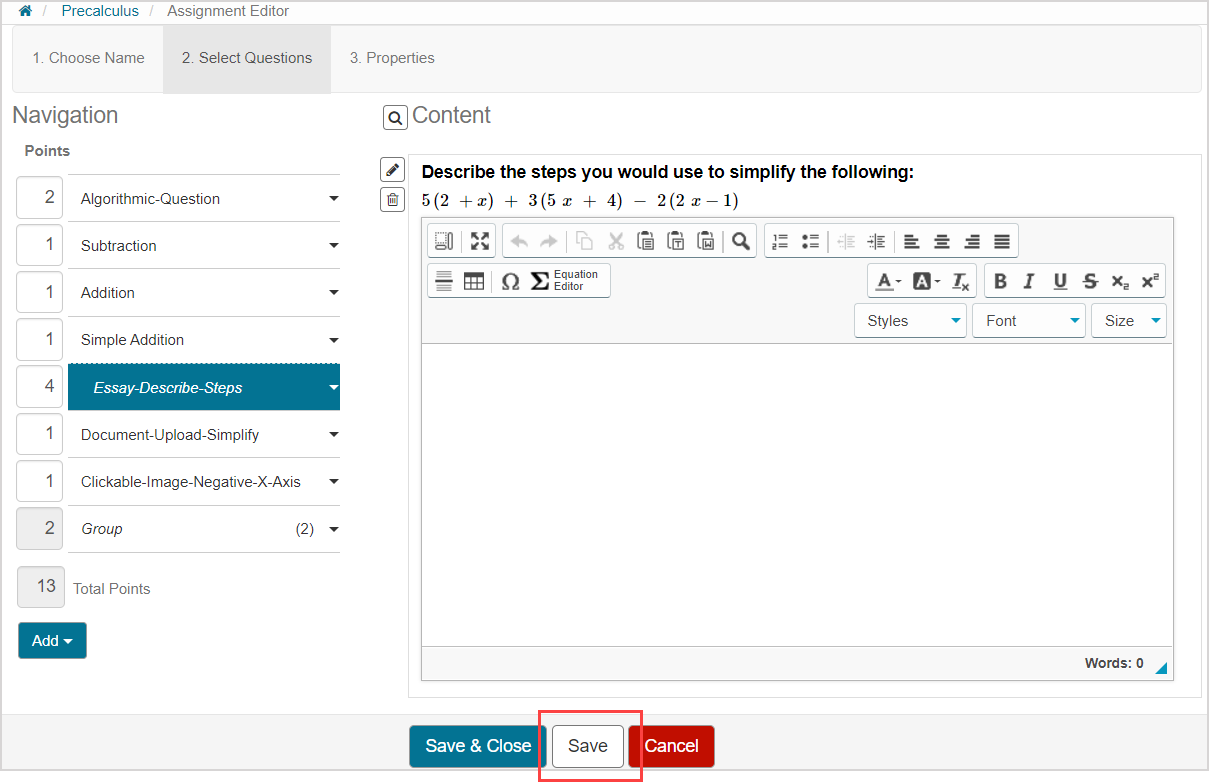TIP: Alternatively, click Save & Close to return to the Content Repository.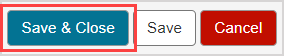1. The new total points value for the assignment is now visible in the Assignment Editor and the student and instructor Gradebooks.